Check Full Chapter Explained - Continuity and Differentiability - Continuity and Differentiability Class 12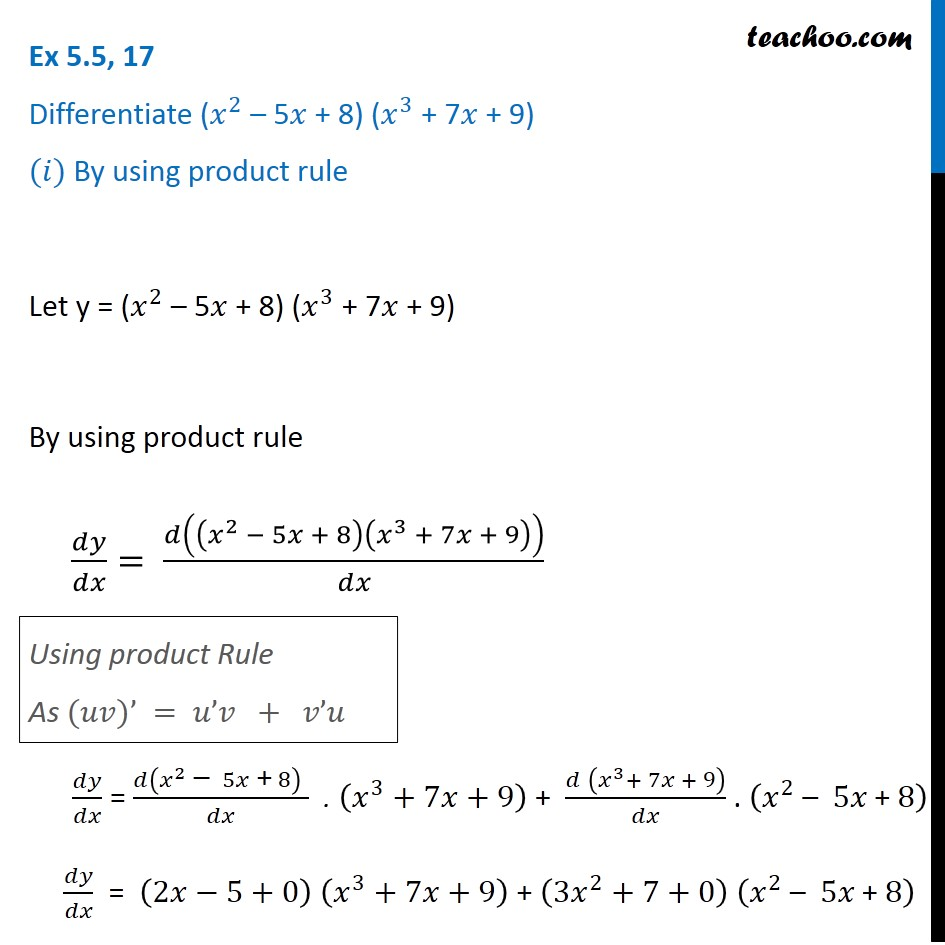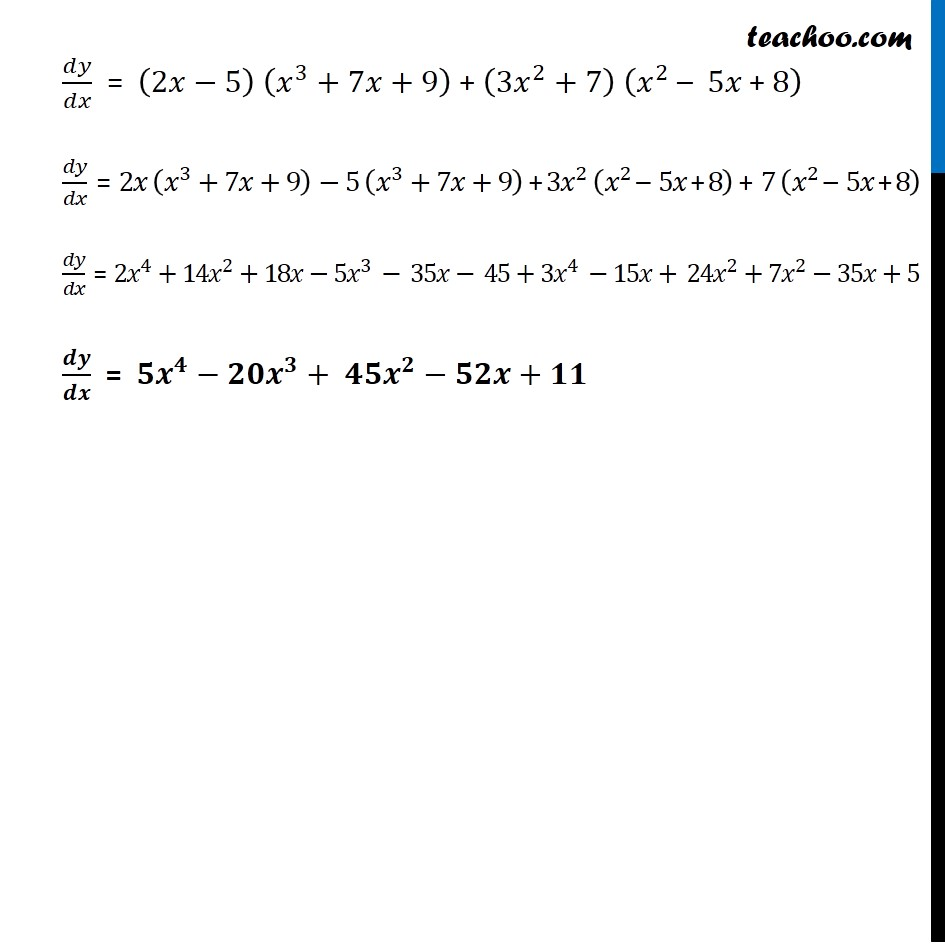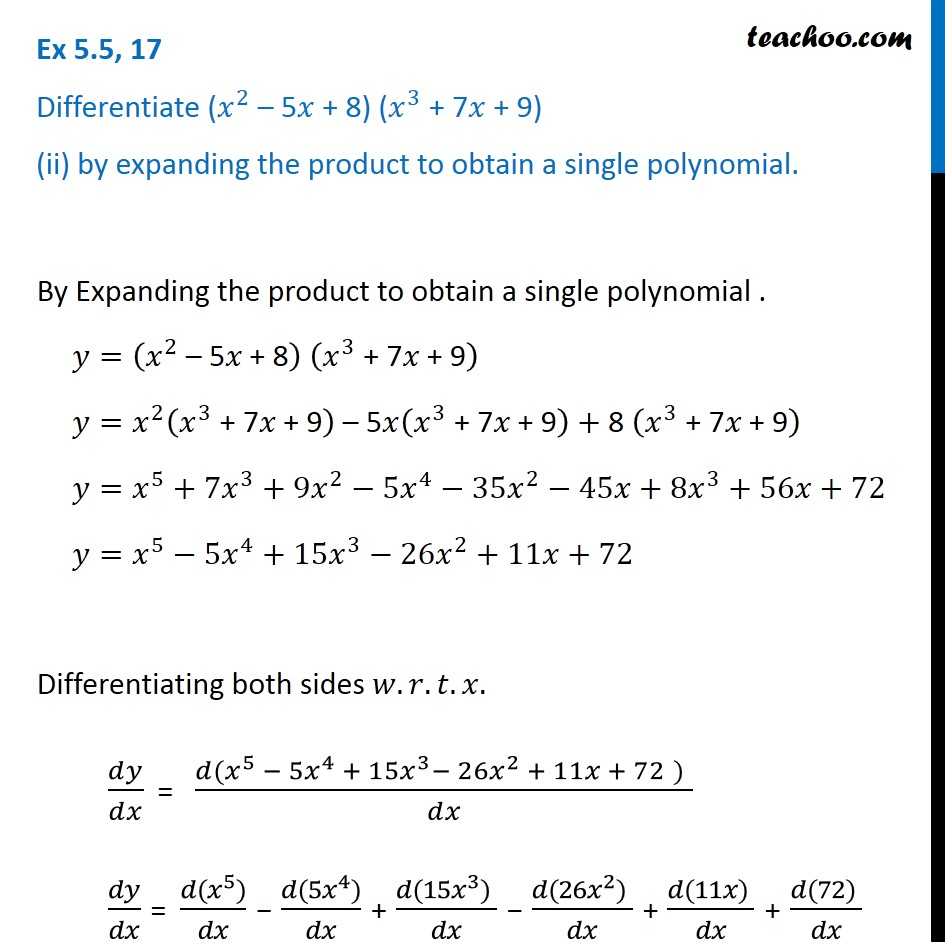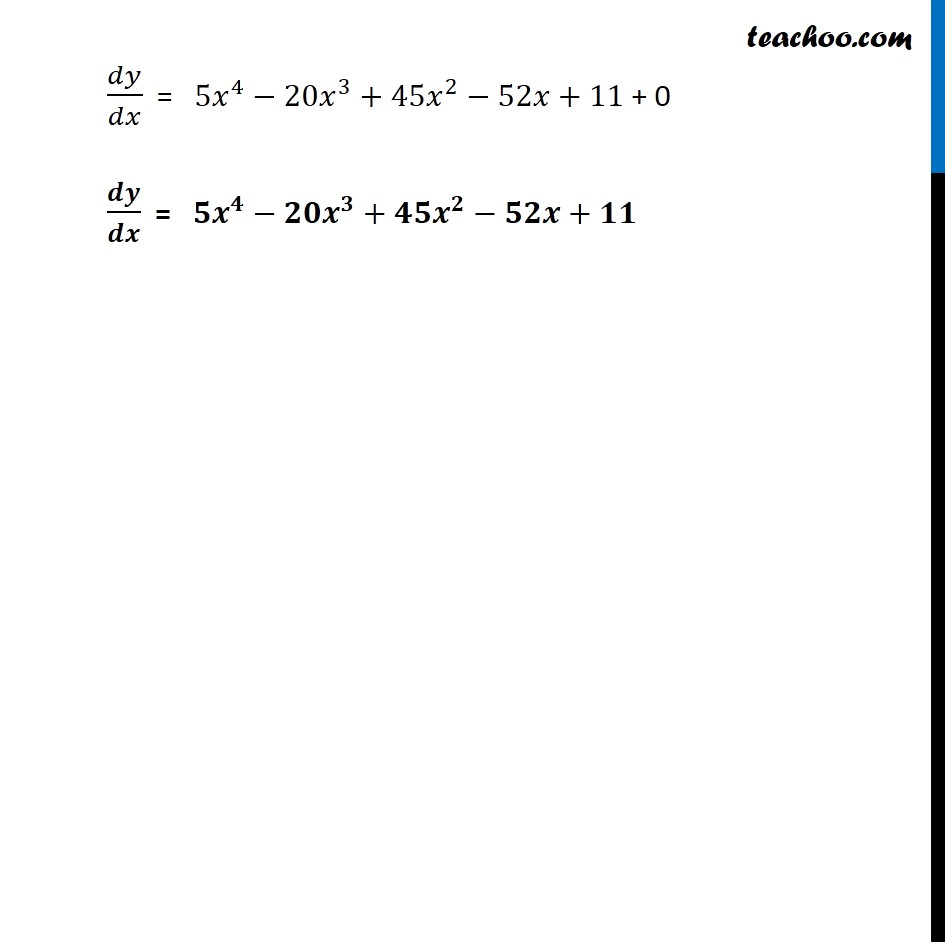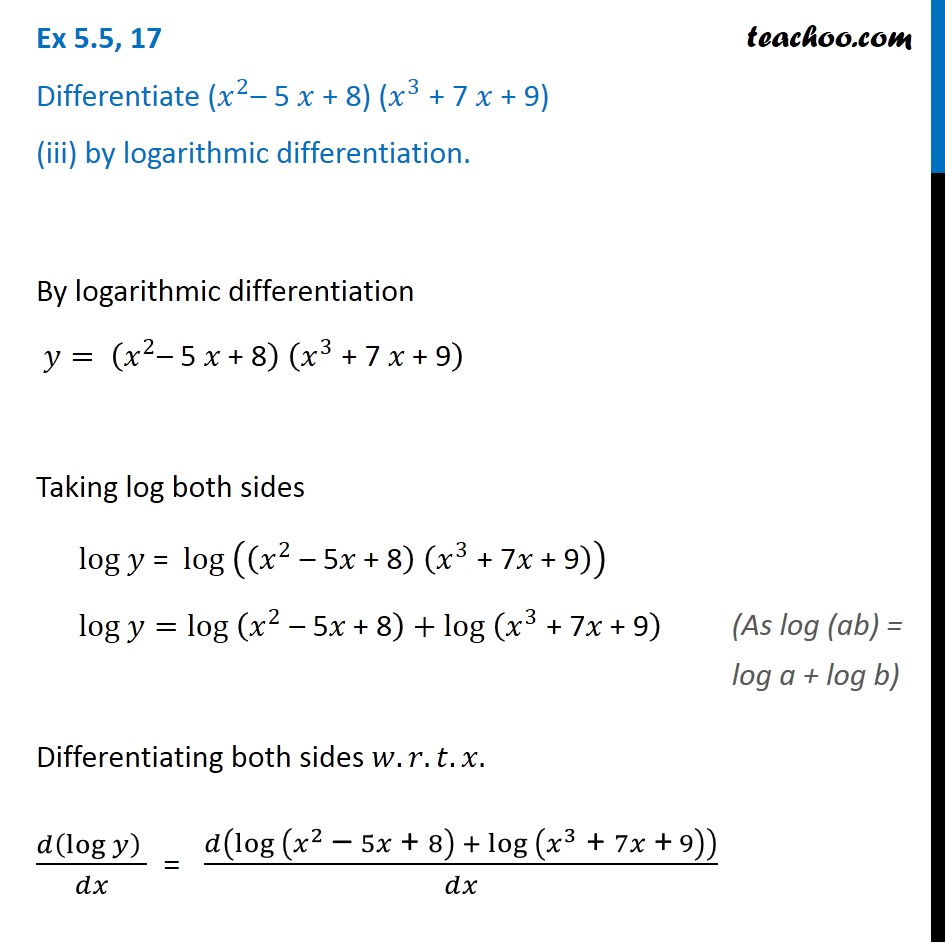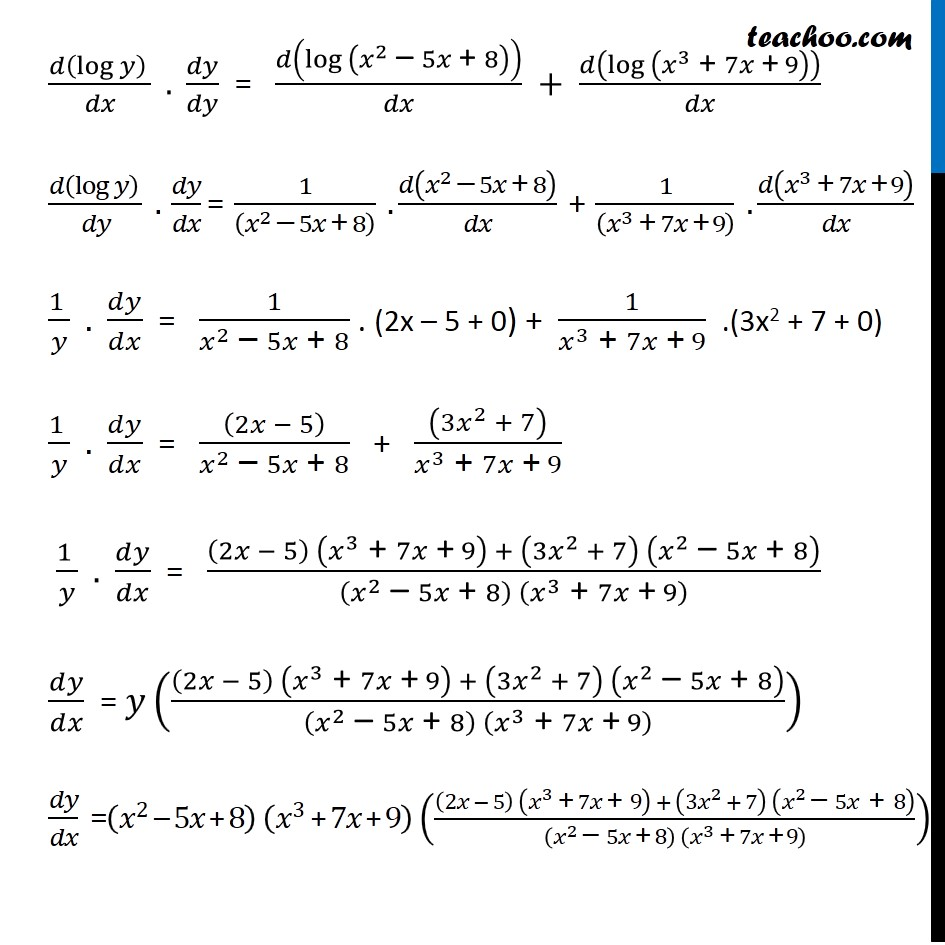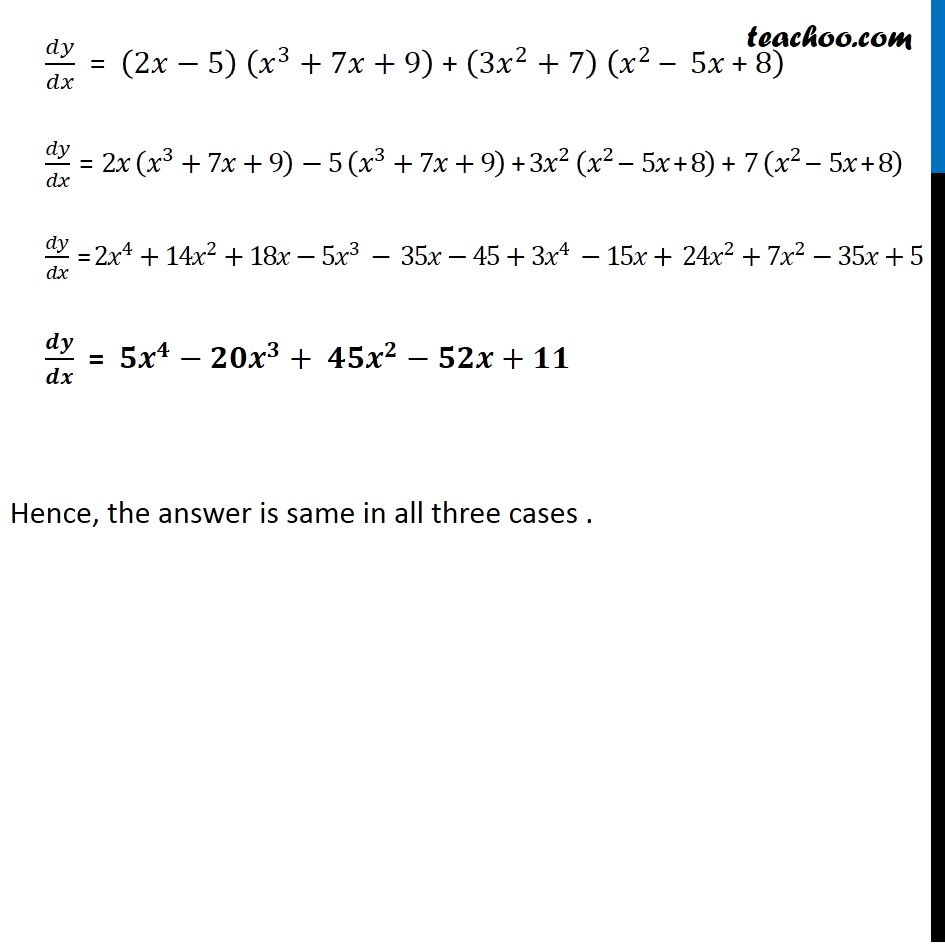1. Chapter 5 Class 12 Continuity and Differentiability
2. Serial order wise
3. Ex 5.5

Transcript

Ex 5.5, 17 Differentiate (𝑥^2 – 5𝑥 + 8) (𝑥^3 + 7𝑥 + 9) (𝑖) By using product rule Let y = (𝑥^2 – 5𝑥 + 8) (𝑥^3 + 7𝑥 + 9) By using product rule 𝑑𝑦/𝑑𝑥= 𝑑((𝑥^2 − 5𝑥 + 8)(𝑥^3 + 7𝑥 + 9))/𝑑𝑥 𝑑𝑦/𝑑𝑥 = 𝑑(𝑥^2 " – " 5𝑥" +" 8)" " /𝑑𝑥 . (𝑥^3+7𝑥+9) + 𝑑" " (𝑥^3+ 7𝑥 + 9)/𝑑𝑥 . (𝑥^2 " – " 5𝑥" +" 8) 𝑑𝑦/𝑑𝑥 = (2𝑥−5+0) (𝑥^3+7𝑥+9) + (3𝑥^2+7+0) (𝑥^2 " – " 5𝑥" +" 8) Using product Rule As (𝑢𝑣)’ = 𝑢’𝑣 + 𝑣’𝑢 𝑑𝑦/𝑑𝑥 = (2𝑥−5) (𝑥^3+7𝑥+9) + (3𝑥^2+7) (𝑥^2 " – " 5𝑥" +" 8) 𝑑𝑦/𝑑𝑥 = 2𝑥 (𝑥^3+7𝑥+9)−5 (𝑥^3+7𝑥+9) + 3𝑥^2 (𝑥^2 " – " 5𝑥" +" 8) + 7 (𝑥^2 " – " 5𝑥" +" 8) 𝑑𝑦/𝑑𝑥 = 2𝑥^4+14𝑥^2+18𝑥−5𝑥^3 − 35𝑥− 45+3𝑥^4 −15𝑥+ 24𝑥^2+7𝑥^2−35𝑥+5 𝒅𝒚/𝒅𝒙 = 𝟓𝒙^𝟒−𝟐𝟎𝒙^𝟑+ 𝟒𝟓𝒙^𝟐−𝟓𝟐𝒙+𝟏𝟏 Ex 5.5, 17 Differentiate (𝑥^2 – 5𝑥 + 8) (𝑥^3 + 7𝑥 + 9) (ii) by expanding the product to obtain a single polynomial. By Expanding the product to obtain a single polynomial . 𝑦=(𝑥^2 " – 5" 𝑥" + 8" ) (𝑥^3 " + 7" 𝑥" + 9" ) 𝑦=𝑥^2 (𝑥^3 " + 7" 𝑥" + 9" )" – 5" 𝑥(𝑥^3 " + 7" 𝑥" + 9" )" + 8 " (𝑥^3 " + 7" 𝑥" + 9" ) 𝑦=𝑥^5+7𝑥^3+9𝑥^2−5𝑥^4−35𝑥^2−45𝑥+8𝑥^3+56𝑥+72 𝑦=𝑥^5−5𝑥^4+15𝑥^3−26𝑥^2+11𝑥+72 Differentiating both sides 𝑤.𝑟.𝑡.𝑥. 𝑑𝑦/𝑑𝑥 = (𝑑(𝑥^5 − 5𝑥^4 + 15𝑥^3− 26𝑥^2 + 11𝑥 + 72" " )" " )/𝑑𝑥 𝑑𝑦/𝑑𝑥 = (𝑑(𝑥^5))/𝑑𝑥 − (𝑑(5𝑥^4))/𝑑𝑥 + (𝑑(15𝑥^3)" " )/𝑑𝑥 − (𝑑(26𝑥^2)" " )/𝑑𝑥 + (𝑑(11𝑥)" " )/𝑑𝑥 + (𝑑(72)" " )/𝑑𝑥 𝑑𝑦/𝑑𝑥 = 5𝑥^4−20𝑥^3+45𝑥^2−52𝑥+11 + 0 𝒅𝒚/𝒅𝒙 = 𝟓𝒙^𝟒−𝟐𝟎𝒙^𝟑+𝟒𝟓𝒙^𝟐−𝟓𝟐𝒙+𝟏𝟏 Ex 5.5, 17 Differentiate (𝑥^2– 5 𝑥 + 8) (𝑥^3 + 7 𝑥 + 9) (iii) by logarithmic differentiation. By logarithmic differentiation 𝑦= (𝑥^2 "– 5 " 𝑥" + 8" ) (𝑥^3 " + 7 " 𝑥" + 9" ) Taking log both sides log 𝑦 = log ((𝑥^2 " – 5" 𝑥" + 8" ) (𝑥^3 " + 7" 𝑥" + 9" )) log 𝑦=log (𝑥^2 " – 5" 𝑥" + 8" )+〖log 〗⁡(𝑥^3 " + 7" 𝑥" + 9" ) Differentiating both sides 𝑤.𝑟.𝑡.𝑥. (𝑑(log⁡𝑦 ) )/𝑑𝑥 = 𝑑(log (𝑥^2 " – " 5𝑥" + " 8) + 〖log 〗⁡(𝑥^3 " + " 7𝑥" +" 9) )/𝑑𝑥 By logarithmic differentiation 𝑦= (𝑥^2 "– 5 " 𝑥" + 8" ) (𝑥^3 " + 7 " 𝑥" + 9" ) Taking log both sides log 𝑦 = log ((𝑥^2 " – 5" 𝑥" + 8" ) (𝑥^3 " + 7" 𝑥" + 9" )) log 𝑦=log (𝑥^2 " – 5" 𝑥" + 8" )+〖log 〗⁡(𝑥^3 " + 7" 𝑥" + 9" ) Differentiating both sides 𝑤.𝑟.𝑡.𝑥. (𝑑(log⁡𝑦 ) )/𝑑𝑥 = 𝑑(log (𝑥^2 " – " 5𝑥" + " 8) + 〖log 〗⁡(𝑥^3 " + " 7𝑥" +" 9) )/𝑑𝑥 (As log (ab) = log a + log b) (𝑑(log⁡𝑦 ) )/𝑑𝑥 . 𝑑𝑦/𝑑𝑦 = 𝑑(log (𝑥^2 " – " 5𝑥" + " 8))/𝑑𝑥 + 𝑑(〖log 〗⁡(𝑥^3 " + " 7𝑥" +" 9) )/𝑑𝑥 (𝑑(log⁡𝑦 ) )/𝑑𝑦 . 𝑑𝑦/𝑑𝑥 = 1/((𝑥^2 " – " 5𝑥" + " 8) ) . 𝑑(𝑥^2 " – " 5𝑥" + " 8)/𝑑𝑥 + 1/((𝑥^3 " + " 7𝑥" +" 9) ) . 𝑑(𝑥^3 " + " 7𝑥" +" 9)/𝑑𝑥 (1 )/𝑦 . 𝑑𝑦/𝑑𝑥 = 1/(𝑥^2 " – " 5𝑥" + " 8) . (2x – 5 + 0) + 1/(𝑥^3 " + " 7𝑥" +" 9) .(3x2 + 7 + 0) (1 )/𝑦 . 𝑑𝑦/𝑑𝑥 = ((2𝑥 − 5))/(𝑥^2 " – " 5𝑥" + " 8) + ((3𝑥^2 + 7))/(𝑥^3 " + " 7𝑥" +" 9) (1 )/𝑦 . 𝑑𝑦/𝑑𝑥 = ((2𝑥 − 5) (𝑥^3 " + " 7𝑥" +" 9) + (3𝑥^2 + 7) (𝑥^2 " – " 5𝑥" + " 8))/((𝑥^2 " – " 5𝑥" + " 8) (𝑥^3 " + " 7𝑥" +" 9) ) 𝑑𝑦/𝑑𝑥 = 𝑦(((2𝑥 − 5) (𝑥^3 " + " 7𝑥" +" 9) + (3𝑥^2 + 7) (𝑥^2 " – " 5𝑥" + " 8))/((𝑥^2 " – " 5𝑥" + " 8) (𝑥^3 " + " 7𝑥" +" 9) )) 𝑑𝑦/𝑑𝑥 =(𝑥^2 " – " 5𝑥" + " 8) (𝑥^3 " + " 7𝑥" +" 9)(((2𝑥 − 5) (𝑥^3 " + " 7𝑥" + " 9) + (3𝑥^2 + 7) (𝑥^2 " – " 5𝑥" + " 8))/((𝑥^2 " – " 5𝑥" + " 8) (𝑥^3 " + " 7𝑥" +" 9) )) 𝑑𝑦/𝑑𝑥 = (2𝑥−5) (𝑥^3+7𝑥+9) + (3𝑥^2+7) (𝑥^2 " – " 5𝑥" +" 8) 𝑑𝑦/𝑑𝑥 = 2𝑥 (𝑥^3+7𝑥+9)−5 (𝑥^3+7𝑥+9) + 3𝑥^2 (𝑥^2 " – " 5𝑥" +" 8) + 7 (𝑥^2 " – " 5𝑥" +" 8) 𝑑𝑦/𝑑𝑥 = 2𝑥^4+14𝑥^2+18𝑥−5𝑥^3 − 35𝑥−45+3𝑥^4 −15𝑥+ 24𝑥^2+7𝑥^2−35𝑥+5 𝒅𝒚/𝒅𝒙 = 𝟓𝒙^𝟒−𝟐𝟎𝒙^𝟑+ 𝟒𝟓𝒙^𝟐−𝟓𝟐𝒙+𝟏𝟏 Hence, the answer is same in all three cases .

Ex 5.5